Courses

# Electronic Control Of DC Shunt Motors Electrical Engineering (EE) Notes | EduRev

## Electrical Engineering (EE) : Electronic Control Of DC Shunt Motors Electrical Engineering (EE) Notes | EduRev

The document Electronic Control Of DC Shunt Motors Electrical Engineering (EE) Notes | EduRev is a part of the Electrical Engineering (EE) Course Electrical Machines.
All you need of Electrical Engineering (EE) at this link: Electrical Engineering (EE)

Electronic Control of DC Shunt Motors
We have seen that in order to use a DC shunt motor, we need separate arrangement for starting it, controlling its speed and stopping it. Now this equipment if we use the conventional equipment is bulky and sometimes also very lossy causing a lot of power loss. Therefore, the modern trend has been to use power electronic devices to control all aspects of operation of a shunt DC motor.

(Refer Slide Time: 01:04)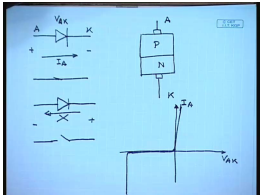Let us see what are these electronic devices? The first device that is used is a simple diode. As you all know a diode is a PN junction semiconductor device. The characteristics of diode are that if you apply a positive voltage between the terminal anode and the terminal cathode, then it behaves like a closed switch with very little voltage drop. But if we apply a reverse polarity of voltage, it behaves like an open switch.

The current through a diode can flow only in one direction from anode to cathode. It cannot flow from cathode to anode; this is not possible. If you look at a practical characteristic of a diode the IV characteristics of a diode that is if you plot voltage V A K versus the current I A, it looks somewhat like this. In the forward direction it almost does not have any voltage drop; in the reverse direction it almost does not carry any current.

(Refer Slide Time: 03:33)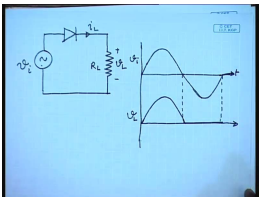So, if I use such a device let us say with a simple AC circuit single phase AC circuit like this; this is current, this is input voltage, this is load current, this is load resistance, this is load voltage. Then this is the input voltage the load voltage. When the input voltage is positive, then this will behave like a closed switch. Hence the input voltage will appear across the load, but when the voltage is negative there will be no voltage. So, this is called a half wave rectifier circuit. While a diode is a useful switch, it cannot control; it cannot be turned on or off by a control signal. That is the conduction interval of the diode will be solely determined by the voltage across it. It cannot be controlled in any other manner there are other switches in which that is possible.

(Refer Slide Time: 05:55)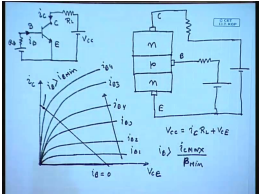For example, the next switch is the known transistor. This is what is called a n p n transistor. This terminal is called collector; this terminal is called base; this terminal is called emitter. You all know that if certain current is injected into the base, then a proportional current flow through the collector terminal. The characteristic of this is also known. We plot i c versus V c E; when there is no base current applied the collector current is 0. So, this transistor behaves like an off switch. As we keep on increasing the base current for a given load resistance R L as we increase the base current the collector current starts increasing and then saturates at a particular value for a given base current determined by the device characteristics.

So, this is i B 1, this is i B 2, this is i B 3, this is i B 4, this is i B 5, and it is increasing in this direction. The load line if this is V c c is given by V c c equal to i c into R L plus V c E which look somewhat like a straight line. Now for this load if the applied base current let us say is larger than I B, then the voltage across the device will be almost 0. So, by knowing what is the maximum current that can flow through the transistor and if we increase i B to be larger than some I c max by what is called the minimum current gain beta minimum, then we can ensure that the drop across the transistor will be very small. And hence, in that case it will act as a closed switch.

So, here we have a switch in which we can control the base current to determine the state of the switch whether it will be off state where corresponds to I b equal to 0 or in the on state corresponding to I b larger than some I b minimum. So, this is another power electronic switch which is a controlled one unlike the diode.

(Refer Slide Time: 10:54)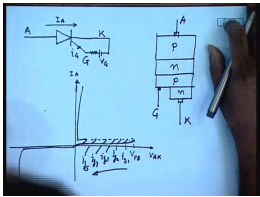The next control switch is called a thyristor. It has also three terminals; like diode it has anode and cathode and like transistor it has another terminal called gate which is the control terminal. In construction it is a PN-PN construction. This is anode; this is cathode, and this is where the gate terminal is, okay. Operation of this device is slightly more complicated, and we will not have occasion to discuss that in detail. It will be sufficient to tell that this is also a controlled switch which can be turned on by injecting a current through the gate terminal. However, in order to turn it off the current flowing through anode and cathode has to be disrupted brought below a certain level, only then it will turn off.

The characteristics look somewhat like this. As you keep on applying forward voltage, the device conducts very little current if there is no gate current up to. Then once it reaches certain maximum voltage called the forward breakdown voltage, then the device turns on; after that it behaves almost like a diode. The reverse side it is like a diode; however, the point at which the device turns on can be controlled by controlling the gate current. Therefore, we can apply different gate current to turn it on at different forward voltage level. So, this is for i g 1, this is for i g 2, this is for i g 3, this is for i g 4, I g 5, etcetera. So, the gate currents increase in this direction.

(Refer Slide Time: 14:16)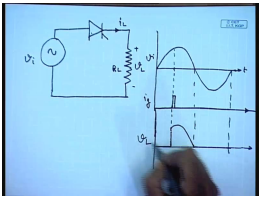So, if we use this kind of a device with our AC circuit with diode, then we find the following waveforms. This is V i, and let us say a gate current is applied at this instant. It is to be understood that it is not necessary to continuously apply the gate current. Once the device has turned off, the gate pulse can be removed and the device will remain on. It can only be turned off when the current through it becomes 0. In that case V L up to this point, this device will behave as an open switch.

And at this point when the large gate current is applied then it will become a closed switch, and afterwards it will follow the input voltage. So, this is how the load voltage will look like. So, these are some the devices which can be used to control the voltage applied to a DC shunt motor and hence control its speed. Now what type of device or circuit will exactly be used depends on what type of supply is available.

(Refer Slide Time: 17:29)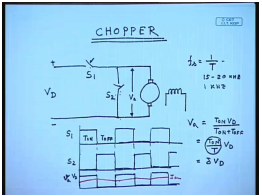If the available supply is DC then the circuit that is used is called chopper. The chopper circuit is very simple. You have one switch here; you have another switch here. These are controlled switches like the transistor, and then you have the DC machine. The pin is normally separately excited. So, let us say this is the input DC voltage. The turn on and turn off of these two switches are complementary; that is when s 1 is on s 2 is off, when s 2 is on s 1 is off. This is necessary; otherwise, if s 1 and s 2 are simultaneously on then there will be a short circuit across the DC supply which must be avoided. So, the on period of s 1 may be something like this. The on period of s 2 will be its logical compliment.

So, what will be the waveform of the voltage applied across the armature? Obviously, whenever s 1 is on the applied voltage across the armature is same as V d. During this period the armature voltage, this is V D. During this period when s 2 is on the applied voltage is 0. So, what is the average armature voltage V a? if this period is T on, this period is T off, then V a equal to average voltage V a equal to T on into V D divided by T on plus T off which comes to T on by T V D. The quantity T on by T is called the duty ratio of the chopper delta equal to delta V D. So, you see this chopper provides a convenient means of controlling the average voltage applied across the DC machine armature terminal.

Now the speed of a DC machine will mostly depend on the average voltage, because this chopping is done at a very high switching frequency. The switching frequency f s equal to 1 by T is usually kept constant, and this can range in the range of 15 to 20 kilohertz for smaller machine. Even for larger machine it will be greater than 1 kilohertz. Now the DC motor armature because it contains winding it also has some amount of inductance. So, if we look at the current waveform even when the applied voltage looks like a pulsed waveform the current it is almost DC; the pulsation in the current is very small. This is I a. Hence, torque is almost smooth, and speed is determined by the average DC voltage. So, what will be the torque speed characteristics of a DC motor driven by such a chopper?

(Refer Slide Time: 22:58)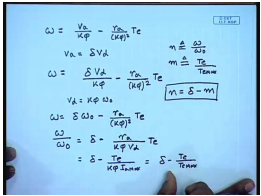We know that the torque speed characteristics of a DC machine is given by omega equal to V a by K phi minus r a by k phi square T e. Now we have seen with a chopper V a equal to delta V d, so omega equal to delta V d by k phi minus r a by k phi square T e. Now let us say the DC machine is rated such that when delta equal to one that is the full voltage V d is applied across its terminal, the DC machine operates at its rated no load speed. So, in other words if we say V d equal to k phi omega 0, where omega 0 is the rated no load speed of the DC machine. Then we can write omega equal to delta omega 0 minus r a by T e, or omega by omega 0 equal to delta minus r a by k phi V d into T e.

But V d by r a is the short circuit I mean direct online starting current of the DC machine. So, this is equal to delta minus T e divided by k phi I a max; that is delta minus T e by T e max which is the maximum torque at direct online starting with a voltage of V d. So, if we define per unit speed n to be equal to omega by omega 0 and per unit of m to be T e by T e max, then the torque speed characteristics is given by the simple equation n equal to delta minus m.

(Refer Slide Time: 26:38)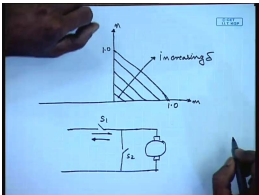That is the torque speed characteristics of a chopper driven DC machine normalized torque speed characteristics is this is n; this is m. These are straight lines 45 degree slope. This is increasing delta. Interesting thing to note is that if these switches can allow average current to flow in the reverse direction; that is instead of current flowing in this direction if it allows in this direction also then the same circuit can be used for regenerative breaking; reason is very simple.

(Refer Slide Time: 28:31)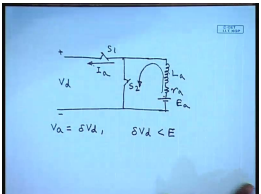If we draw the transient equivalent circuit of the DC machine we will find that in addition to resistance it has an armature inductance. This is the back E m f. This is called the L a, r a, E a. So, whenever s 2 is turned on the current flows through the armature inducting in this way, and when it is turned off then the inductor current cannot vanish immediately. Hence, it starts flowing through the switch s 1. So, we see if this switch is still on then you can send the current back to the supply. When will this current be reserved? we have seen the terminal voltage V a equal to V d delta V d. So, if delta V d is less than the induced back E m f be it E, then of course, the direction of the current will change and hence it can be used for regenerative breaking purpose.

(Refer Slide Time: 30:30)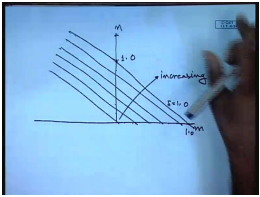Therefore, the torque speed characteristic of such a chopper driven DC machine is the same on both the quadrants. So, one advantage we see of using a chopper that regionality breaking is now possible even below base speed. This is n equal to 1.0 corresponding to the base speed. This is because the effective voltage applied across the DC machine can be controlled by controlling the duty cycle delta. This is when a chopper is DC supply is available and a chopper is used, but more often the not the available supply is AC.

(Refer Slide Time: 32:22)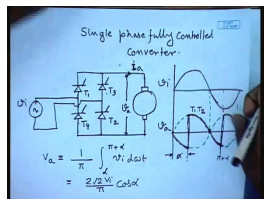So, if we have a single phase AC then we can use another power electronic convertor called the single phase fully controlled convertor. In this you have the convertor looks like a diode rectifier bridge, but instead of diode we use thyristors. This is say T 1, this is T 2, this is T 3, this is T 4. This is the AC source and the DC machine is connected here. Let us say this is the input voltage V I, had this been diodes we know that the voltage waveform would have been a full wave rectifier sin wave. But since this is the thyristor, the full wave rectifier sin wave is not possible. So, we delay the firing of the thyristor T 1 with respect to the zero crossing of the supply by an angle let us say alpha which is called the firing angle.

Then the voltage starts, the V a starts following the rectifier sign wave from this point; however, as we have mentioned that this armature has inductance. So, even though the armature voltage becomes zero here the current through the armature may not be zero, and hence it will continue to follow the T 1 T 2 will then not turn off, because until and unless current through them becomes 0; these devices will not turn off. So, it will continue to follow the input voltage V i. And if the current does not becomes zero, before the next set of thyristor that is T 3 T 4 here, T 1 and T 2 are fired firing that is gate pulses applied to them. So, it will continue up to the point where T 3 and T 4 are gated.

When T 3 and T 4 are gated, what will happen? This T 1 which was normally on switch, when T 3 is turned on then a reverse voltage V i which is now positive. Well, minus V i will be applied across T 1 and the current through it will transfer to T 3; that way T 1 will turn off. Hence, now the armature voltage will start following minus here; same thing will happen here. So, the final armature voltage will look somewhat like this. As we have seen that the speed of the DC machine depends on the average armature voltage. So, it will be imperative to find out what is the average armature voltage here V a equal to 1 by, since it is symmetric over one cycle it will be sufficient to integrate over onehalf cycle. This comes out to be, where V i is the R m s of the input voltage.

(Refer Slide Time: 38:36)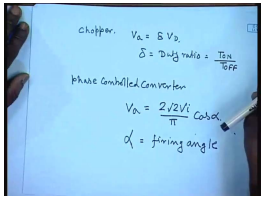So, you have considered two power electronic convertors to control the average DC voltage applied across the armature of a DC shunt motor. For chopper average applied voltage V a equal to delta V d where delta is the duty ratio equal to T on by T off for phase control convertor. The second one V a equal to 2 root 2 V i by pi cos alpha which is alpha is called the firing angle. Unfortunately, for this case though there is no way this armature current can flow in the opposite direction.

So, degenerative breaking in the forward direction is not possible, but we can see that if we make alpha larger than 90 degree then the average DC voltage becomes negative in which case when the current flows in this direction, then with this terminal positive and this terminal negative the power is still fed back to the supply. Hence regenerative breaking is possible, but for that the direction of rotation of the motor must reverse; that is the back E m f must reverse. So, let us try to solve one or two problems to see how the speed of the machine can be controlled using single phase fully controlled convertor.

(Refer Slide Time: 41:00)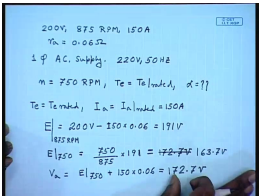So, let us say we have a DC machine whose rated terminal voltage is 200 volts, speed is 875 RPM, and armature current is 150 amperes. The armature resistance r a equal to 0.06 ohm. Now it is supplied from a single phase AC supply source voltage 220 volt 50 hertz. Let us say we want to operate the machine at 750 RPM, and torque should be equal to T e rated; find out what should be the firing angle alpha? So, under rated operation when T e equal to T e rated assuming rated flux I a equal to I a rated equal to 150 amperes, then what is E? E equal to rated terminal voltage which is 200 volts minus at a rated speed that is at 870 RPM, because that is when 200 volt is applied minus I a which is 150 amperes into armature resistance 0.06 ohms. This comes to 191 volts.

So, at E at f 750 RPM, how much it will be? This is 750 by 875 into 191 equal to 172.5 volts. So, what if we now want to operate the machine at the rated torque, at 750 RPM what should be the terminal voltage average armature voltage V a should be? E 750 plus rated armature current 150 amperes into armature resistance. Sorry, this comes to 163.7 volts; this comes to 172.7 volts.

(Refer Slide Time: 45:19)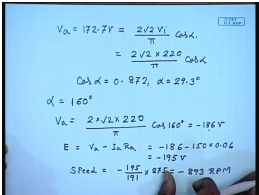Now this V a is equal to 2 root 2 V i by pi cos alpha equal to 2 root 2 into 220 by pi cos alpha. This gives cos alpha equal to 0.872 or alpha equal to 29.3 degrees. So, almost at alpha equal to 30 degree we will be able to reduce the motor speed to 75 RPM while still supplying rated torque. Now looked at the other way, let us say now we have increased this alpha to 160 degree, but it is still developing rated torque. So, what will be the motor speed in that case? So, at alpha equal to 160 V a equal to 2 into root 2 into 220 by pi cos 160 degree. This comes to minus 186 volts. Then E is V a minus I a R a.

Please note that in a phase controlled convertor, the current can flow only in one direction; that is it can flow only into the mode of terminal. So, this comes to minus 186 minus 150 into 0.6; this comes to minus 195 volts. So, the speed will be approximately minus 893 RPM. So, we see with alpha larger than 90 degree, the motor operates in the opposite direction and works in the degenerating breaking port, because the terminal voltage is negative; the current is positive. Hence, power is fed back to the DC supply. Let us try to solve similar problems involving choppers.

(Refer Slide Time: 49:34)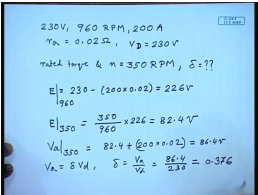So, we have a 230 volt, 960 RPM, 200 ampere separately excited DC motor, it is r a equal to 0.02 ohm. This motor is fed from a chopper, whereas supply voltage V d equal to 230 volt. If I want to operate this motor at a rated torque and a speed equal to 350 RPM, then what should be the delta? Under rated condition of the machine we know E equal to 230 minus. So, this is E at 960 RPM; so E at 350 RPM. So, V a at 350 RPM and rated torque this will be 82.4 plus, at rated torque the current is also rated. So, 200 into 0.02; this comes to 86.4 volt. We know V a equal to delta V d or delta equal to V a by V d equal to 86.4 by 230. So, this delta will be 0.376. So, it will be interesting also to find out what is the maximum control of speed control of such an arrangement?

(Refer Slide Time: 52:40)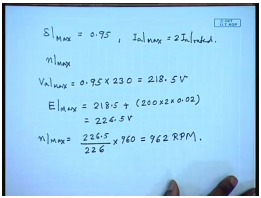For that let us assume that maximum value of delta has certain limit 0.95. This comes because the switches which are power electronic switches require a minimum on time and off time. So, delta cannot really be made 1 or 0. So, under this condition and let us say the maximum permissible two times I a rated. So, calculate the maximum possible speed without resorting to field weakening. Now since delta its maximum value is 0.95, then V a maximum is 0.95 into 230 equal to 218.5 volts.

Please note that the speed in the regenerative breaking mode is higher than the motoring mode; therefore, maximum N will occur in the regenerative breaking mode. In that case the current will flow out of the motor terminal. So, E max equal to V max which is 218.5 volts plus maximum armature current which is two times the rated current of 200 into armature resistance. This comes to 226.5 volt. So, n max is E max that is 226.5 by E rated into rated speed. So, this is 962 RPM.

Thank you.

Offer running on EduRev: Apply code STAYHOME200 to get INR 200 off on our premium plan EduRev Infinity!

61 docs|20 tests

,

,

,

,

,

,

,

,

,

,

,

,

,

,

,

,

,

,

,

,

,

;